# Abstract Algebra : Rings

## Example Questions

### Example Question #1 : Abstract Algebra

Which of the following is an ideal of a ring?

Minimum Ideal

All are ideals of rings.

Prime Ideal

Multiplicative Ideal

Associative Ideal

Prime Ideal

Explanation:

When dealing with rings there are three main ideals

Proper Ideal: Whenis a commutative ring, andis a non empty subset ofthen,is said to have a proper ideal if both the following are true.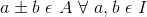and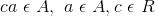Prime Ideal: Whenis a commutative ring,is a prime ideal if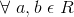is true and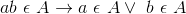Maximal Ideal: Whenis a commutative ring, andis a non empty subset ofthen,has a maximal ideal if all idealare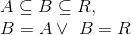Looking at the possible answer selections, Prime Ideal is the correct answer choice.

### Example Question #6 : Abstract Algebra

Which of the following is an ideal of a ring?

Associative Ideal

None are ideals

Maximal Ideal

Communicative Ideal

Minimal Ideal

Maximal Ideal

Explanation:

When dealing with rings there are three main ideals

Proper Ideal: Whenis a commutative ring, andis a non empty subset ofthen,is said to have a proper ideal if both the following are true.andPrime Ideal: Whenis a commutative ring,is a prime ideal ifis true andMaximal Ideal: Whenis a commutative ring, andis a non empty subset ofthen,has a maximal ideal if all idealareLooking at the possible answer selections, Maximal Ideal is the correct answer choice.

### Example Question #7 : Abstract Algebra

Which of the following is an ideal of a ring?

Proper Ideal

Associative Ideal

Minimal Ideal

Communicative Ideal

All are ideals

Proper Ideal

Explanation:

When dealing with rings there are three main ideals

Proper Ideal: Whenis a commutative ring, andis a non empty subset ofthen,is said to have a proper ideal if both the following are true.andPrime Ideal: Whenis a commutative ring,is a prime ideal ifis true andMaximal Ideal: Whenis a commutative ring, andis a non empty subset ofthen,has a maximal ideal if all idealareLooking at the possible answer selections, Prime Ideal is the correct answer choice.

### All Abstract Algebra Resources